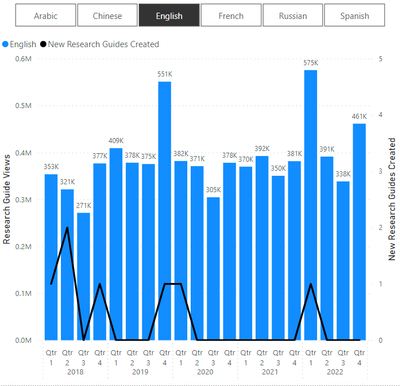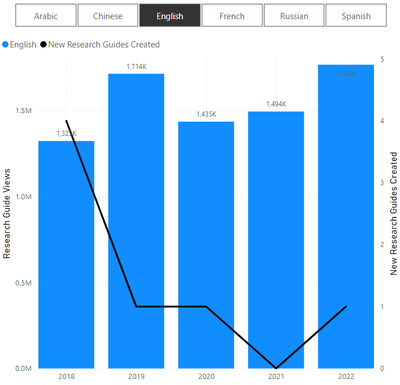cancel
Showing results for
Did you mean:Regular Visitor

## Maximum of measure aggregated dynamically at different date hierarchy levels

Hi community, I have an interesting problem that I'm not able to find a solution for anywhere here:

• I have a measure calculating the sum of a value, let's say [measure] = SUM([value])
• I am putting this measure on the y-axis of a bar chart, where the x-axis is the date hierarchy for a date field, [date]
• Users can drill up or expand down the x-axis date hierarchy, e.g. from quarter-level to year-level
• I want to create a measure (or is it a column?) that will return the maximum of [measure] as displayed in the visual, meaning that it needs to be dynamic based off the date hierarchy level specified in the visual

Here is the bar chart below, with [measure] on the secondary-axis as a black line:In the above, the date hierarchy level is quarter, and at this specified level, the max of [measure] is 2, therefore I want my desired measure to return 2. In the below however, the date level is year, and here I want my desired measure to return 4.Note also that my desired measure also needs to be dynamic based off of slicer filtering - in this case, for language.

Is this possible?

2 REPLIES 2Super User

@daweedle , Based on what I got

Switch( True() ,

isinscope(Date[Date]) , [M1],

isinscope(Date[Month]) , [M2],

isinscope(Date[Qtr]) , [M3],

[M4]

)Regular Visitor

This does not work. My desired measure needs to return the maximum at the grand total level, i.e. the maximum value of [measure] for the whole data set (with filtering).

The challenge is that I require a 2-step calculation. The 1st calculation is for [measure], which is calculated at different levels as per user-selected hierarchy level. The 2nd calculation is performed at the grand total level, to get the max value of [measure] across all values returned from the 1st calculation. Using ISINSCOPE will return a blank for the 2nd calculation since it will return FALSE at the 2nd calculation.

MAXX(SUMMARIZE(Table, [DateLevel], "M", [measure]), [M])

where [DateLevel] is dynamic and specified by the date level of the visual. ISINSCOPE does allow me to check the date level but only for calculations at that same level - and unfortunately not for calculating at a higher level to get the aggregate maximum.